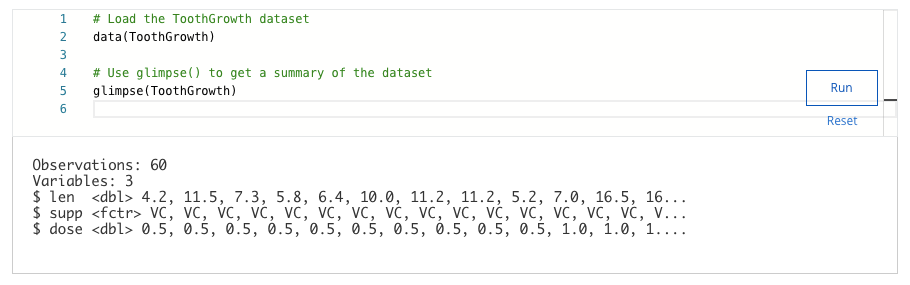## Week 3 – Working with data in R – Shuffle Q/A 2

#### 25. A data analyst is working with a data frame called salary_data. They want to create a new column named hourly_salary that includes data from the wages column divided by 40. What code chunk lets the analyst create the hourly_salarycolumn?

• mutate(salary_data, hourly_salary = wages / 40)
• mutate(salary_data, hourly_salary = wages * 40)
• mutate(hourly_salary = wages / 40)
• mutate(hourly_salary, salary_data = wages / 40)

#### 26. In R, which statistical measure demonstrates how strong the relationship is between two variables?

• Correlation
• Maximum
• Standard deviation
• Average

#### 27. A data analyst creates two different predictive models for the same dataset. They use the bias() function on both models. The first model has a bias of -40. The second model has a bias of 1. Which model is less biased?

• The second model
• It can’t be determined from this information
• The first model

#### 28. What scenarios would prevent you from being able to use a tibble?

• You need to create column names
• You need to store numerical data
• You need to create row names
• You need to change the data types of inputs

#### 29. A data analyst is working with a data frame named salary_data. They want to create a new column named wagesthat includes data from the rate column multiplied by 40. What code chunk lets the analyst create the wages column?

• mutate(salary_data, wages = rate * 40)
• mutate(salary_data, wages = rate + 40)
• mutate(wages = rate * 40)
• mutate(salary_data, rate = wages * 40)

• bias()
• cor()
• sd()
• mean()

#### 31. A data analyst is considering using tibbles instead of basic data frames. What are some of the limitations of tibbles? Select all that apply.

• Tibbles can overload a console
• Tibbles can never change the input type of the data
• Tibbles won’t automatically change the names of variables
• Tibbles won’t automatically change the names of variables

#### 32. A data analyst wants a high level summary of the structure of their data frame, including the column names, the number of rows and variables, and type of data within a given column. What function should they use?

• colnames()
• rename_with()
• str()

#### 33. You are working with the ToothGrowth dataset. You want to use the glimpse() function to get a quick summary of the dataset. Write the code chunk that will give you this summary.• 5
• 4
• 2
• 3

#### 34. A data analyst is working with the penguins dataset in R. What code chunk will allow them to sort the penguins data by the variable bill_length_mm?

• arrange(penguins, bill_length_mm)
• arrange(bill_length_mm, penguins)
• arrange(=bill_length_mm)
• arrange(=bill_length_mm)

## Shuffle Q/A 2

#### 35. A data analyst is working with a data frame called sales. In the data frame, a column named location represents data in the format “city, state”. The analyst wants to split the city into an individual city column and state into a new countrycolumn. What code chunk lets the analyst split the location column?

• separate(sales, location, into=c(“country”, “city” ), sep=”, “)
• separate(sales, location, into=c(“city”, “country”), sep=”, “)
• untie(sales, location, into=c(“city”, “country”), sep=”, “)
• separate(sales, location, into=c(“country”, “city” ), sep=” “)